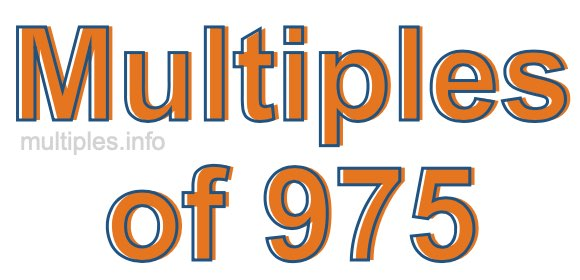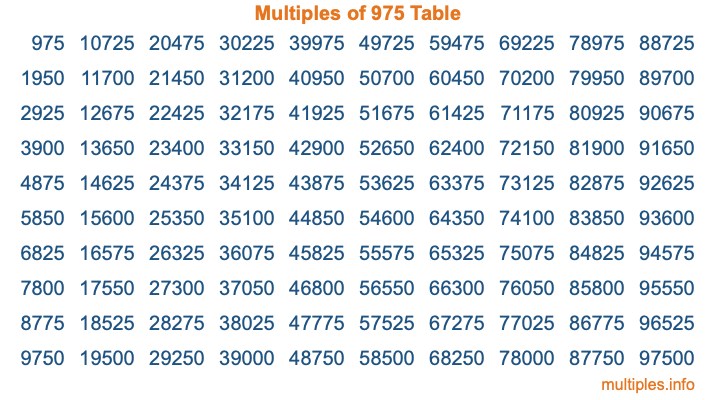Multiples of 975Welcome to the Multiples of 975 page. Here we will first teach you everything you will ever need to know about the multiples of 975, and then give you a study guide summary of everything we taught you to make sure you remember it all. Use this page to look up facts and learn information about the multiples of 975. This page will make you a multiples of nine hundred seventy-five expert!

Definition of Multiples of 975
Multiples of 975 are all the numbers that when divided by 975 equal an integer. Each of the multiples of 975 are called a multiple. A multiple of 975 is created by multiplying 975 by an integer.

Therefore, to create a list of multiples of 975, you start with 1 multiplied by 975, then 2 multiplied by 975, then 3 multiplied by 975, and so on for as long as you want. Thus, the list of the first five multiples of 975 is 975, 1950, 2925, 3900, and 4875. To see a larger list of multiples of 975, see the printable image of Multiples of 975 further down on this page. We also have a category where you can choose any nth multiple of 975.

Multiples of 975 Checker
The Multiples of 975 Checker below checks to see if any number of your choice is a multiple of 975. In other words, it checks to see if there is any number (integer) that when multiplied by 975 will equal your number. To do that, we divide your number by 975. If the the quotient is an integer, then your number is a multiple of 975.

Is  a multiple of 975?

Least Common Multiple of 975 and ...
A Least Common Multiple (LCM) is the lowest multiple that two or more numbers have in common. This is also called the smallest common multiple or lowest common multiple and is useful to know when you are adding our subtracting fractions. Enter one or more numbers below (975 is already entered) to find the LCM.

Check out our LCM Calculator if you need more details about the Least Common Multiple or if you need the LCM for different numbers for adding and subtraction fractions.

nth Multiple of 975
As we stated above, 975 is the first multiple of 975, 1950 is the second multiple of 975, 2925 is the third multiple of 975, and so on. Enter a number below to find the nth multiple of 975.

th multiple of 975

Multiples of 975 vs Factors of 975
975 is a multiple of 975 and a factor of 975, but that is where the similarities end. All postive multiples of 975 are 975 or greater than 975. All positive factors of 975 are 975 or less than 975.

Below is the beginning list of multiples of 975 and the factors of 975 so you can compare:

Multiples of 975: 975, 1950, 2925, 3900, 4875, etc.

Factors of 975: 1, 3, 5, 13, 15, 25, 39, 65, 75, 195, 325, 975

As you can see, the multiples of 975 are all the numbers that you can divide by 975 to get a whole number. The factors of 975, on the other hand, are all the whole numbers that you can multiply by another whole number to get 975.

It's also interesting to note that if a number (x) is a factor of 975, then 975 will also be a multiple of that number (x).

Multiples of 975 vs Divisors of 975
The divisors of 975 are all the integers that 975 can be divided by evenly. Below is a list of the divisors of 975.

Divisors of 975: 1, 3, 5, 13, 15, 25, 39, 65, 75, 195, 325, 975

The interesting thing to note here is that if you take any multiple of 975 and divide it by a divisor of 975, you will see that the quotient is an integer.

Multiples of 975 Table
Below is an image of the first 100 multiples of 975 in a table. The table is in chronological order, column by column. The first column has the first ten multiples of 975, the second column has the next ten multiples of 975, and so on.The Multiples of 975 Table is also referred to as the 975 Times Table or Times Table of 975. You are welcome to print out our table for your studies.

Negative Multiples of 975
Although not often discussed or needed in math, it is worth mentioning that you can make a list of negative multiples of 975 by multiplying 975 by -1, then by -2, then by -3, and so on, to get the following list of negative multiples of 975:

-975, -1950, -2925, -3900, -4875, etc.

Multiples of 975 Summary
Below is a summary of important Multiples of 975 facts that we have discussed on this page. To retain the knowledge on this page, we recommend that you read through the summary and explain to yourself or a study partner why they hold true.

There are an infinite number of multiples of 975.

A multiple of 975 divided by 975 will equal a whole number.

975 divided by a factor of 975 equals a divisor of 975.

The nth multiple of 975 is n times 975.

The largest factor of 975 is equal to the first positive multiple of 975.

975 is a multiple of every factor of 975.

975 is a multiple of 975.

A multiple of 975 divided by a divisor of 975 equals an integer.

975 divided by a divisor of 975 equals a factor of 975.

Any integer times 975 will equal a multiple of 975.

Multiples of a Number
Here you can get the multiples of another number, all with the same attention to detail as we did for multiples of 975 on this page.

Multiples of
Multiples of 976
Did you find our page about multiples of nine hundred seventy-five educational? Do you want more knowledge? Check out the multiples of the next number on our list!